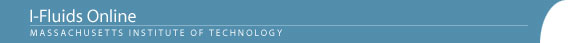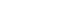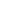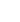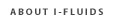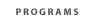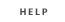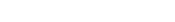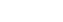2.25: Advanced Fluid Dynamics Section 1: Continuum viewpoint and the equation of motion Section 2: Static Fluids Section 3: Mass Conservation Section 4: Inviscid flow - differential approach Section 5: Control Volume Theorums Section 6: Navier-Stokes equation and viscous flow Section 7: Similarity and dimensional analysis Section 8: Boundary layers, separation and effect on drag/lift Section 9: Vorticity and circulation Section 10: Potential flows; lift, drag and thrust production Section 11: Surface tension and its effect on flows Section 12: Introduction to turbulence Back to 2.25 HomeSection 2: Static Fluids2.1 Equation for pressure distribution in a fluid which is static in some reference frame (its "rest-frame”).
2.2 Boundary condition on pressure at a density discontinuity in the absence of surface tension.
2.3 Pressure distribution in a uniform gravitational field of fluid with constant density. Manometers and barometers, forces on dams and gates, buoyancy forces on submerged and floating bodies, hot-air balloons. Stability.
2.4 Compressible fluids in static equilibrium in a uniform gravitational field. The isothermal ideal-gas model of the atmosphere, and the constant-compressibility model of the ocean.
2.5 Pressure distribution in accelerating rest-frames. Liquids at rest in linearly accelerating and rotating reference frames; pressure distribution in a gas centrifuge.

Reading

Fay, Chapter 2, pages 44-75

Problem Set Chapter 2
Problem 1.3
Problem 1.4
Problem 1.7
Problem 1.8
Problem 1.9
Problem 1.10
Problem 1.11
Problem 1.18